# 基于CNN的人群密度图估计方法简述

1. 传统的人群计数方法
传统的人群计数方法可以分为两类，基于检测的方法和基于回归的方法。

• 基于检测的方法
早期的计数方法主要是基于检测的方法，使用一个滑动窗口来检测场景中的人群，并统计人数。 基于检测的方法可以分为两类：

• 基于整体的检测，训练一个分类器，利用从行人全身提取到的小波，HOG，边缘等特征检测行人。 学习的方法有SVM，boosting和随机森林等。 基于整体的方法主要适用于稀疏人群，随着人群密度的提升，人与人之间的遮挡越来越严重，这种方法就不再适用。
• 基于部分的身体的检测。 为了处理人与人的遮挡问题，主要通过检测身体的部分结构，例如头，肩膀等去统计人群的数量。这种方法比之基于整体的检测，在效果上有略微的提升。
• 基于回归的方法
论何种基于检测的方法，都很难处理人群之间严重的遮挡问题。所以，基于回归的方法逐渐被用来解决人群计数的问题。 基于回归的方法，主要思想是学习一种特征到人群数量的映射。该类方法主要分为两步：1. 提取场景的低级特征，例如例如前景特征，边缘特征，纹理和梯度特征；2. 学习一个回归模型，例如线性回归，岭回归或者高斯过程回归，学习一个低级特征到人群数的映射关系。

• 基于密度图的方法
基于回归的方法虽然能在一定程度上解决遮挡的问题，但是由于是使用整幅图像的特征进行回归技术，从而忽略了图像的空间信息。在2010年的Visual Geometry Group University of Oxford提出了：学习图像的局部特征和其相应的密度图之间的映射，从而在计数的过程中加入图像的空间信息。 论文地址：Learning To Count Objects in Images . 该论文最早提出使用密度图来进行计数的方法，后来很多的图像计数多数基于此方法。

2. 基于深度学习的方法

### MCNN (cvpr2016)

zhang等人提出了 Multi-column Convolutional Neural Network (MCNN)网络来预测人群的密度图。MCNN中有三大创新：

• 提出了MCNN网络，能够处理任意大小的图像，并且使利用3个具有不同卷积核大小的网络来分别提取人群图像的特征，来适应人群头部大小的变化。
• 提出一种根据人头标记生成人群密度图的方法
• 构建了一个新的数据集 Shanghaitech ，包含1198张图像约有330000个人头标记数据。

1. 前景的分割，现有的计数方法通常需要前景分割，而前景分割是很困难的。使用MCNN网络则不需要进行前景分割，输入任意的人群图像，通过CNN网络直接估计其密度图。
2. 密集人群存在严重的遮挡
3. 人群图像中人的尺度存在着较大的差异就需要融合多种图像的特征来估计人群的数量，这些特征人工则很难设计。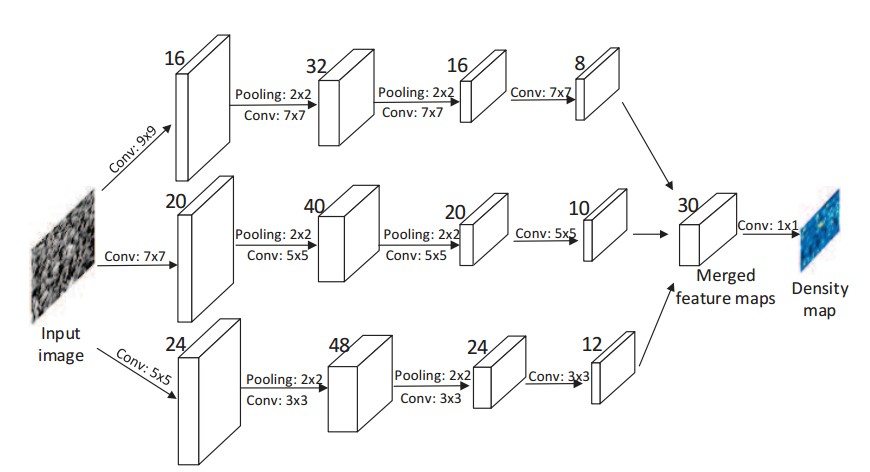#### 密度图的生成

img = cv2.imread(path)
density = np.zeros((img.shape,img.shape))

for i inr range(0,len(gt)):
if int(gt[i]) < img.shape and int(gt[i]) < img.shape:
density[int(gt[i]),int(gt[i])] = 1
density = cv2.GaussianBlur(density,(5,5))


    gt_count = np.count_nonzero(gt)
if gt_count == 0:
return density

# FInd out the K nearest neighbours using a KDTree
pts = np.array(list(zip(np.nonzero(gt).ravel(), np.nonzero(gt).ravel())))
leafsize = 2048

# build kdtree
tree = scipy.spatial.KDTree(pts.copy(), leafsize=leafsize)

# query kdtree
distances, locations = tree.query(pts, k=4)

for i, pt in enumerate(pts):
pt2d = np.zeros(gt.shape, dtype=np.float32)
pt2d[pt,pt] = 1.
if gt_count > 1:
sigma = (distances[i]+distances[i]+distances[i])*0.1
else:
sigma = np.average(np.array(gt.shape))/2./2. #case: 1 point

#Convolve with the gaussian filter
density += scipy.ndimage.filters.gaussian_filter(pt2d, sigma,             mode='constant')


### CP-CNN (cvpr 2017)

CP-CNN( Generating High-Quality Crowd Density Maps using Contextual Pyramid CNNs) 使用图像的全局和局部特征信息生成估计人群图像的密度图。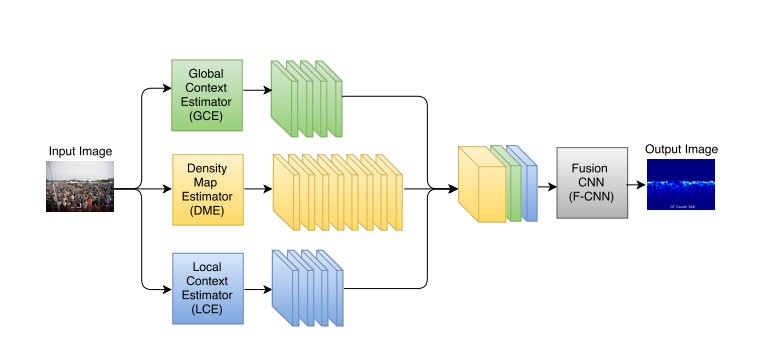### CSRNet (cvpr 2018)

CSRnet网络模型主要分为前端和后端网络，采用剔除了全连接层的VGG-16作为CSRnet的前端网络，提取图像的特征，输出密度图的大小为原始输入图像的1/8。采用空洞卷积神经网络作为后端网络，在保持分辨率的同时扩大感知域， 生成高质量的人群分布密度图。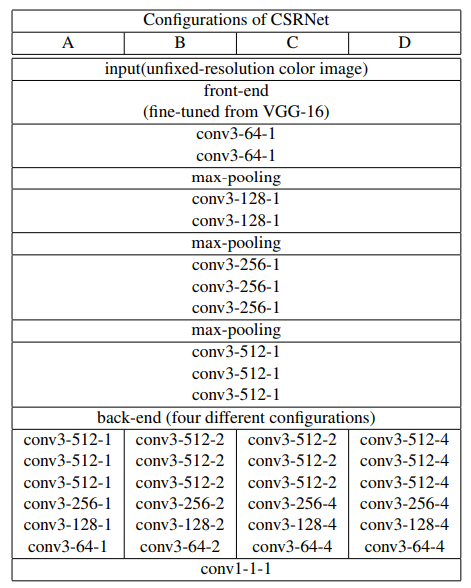CSRNet的主要思路是，使用预训练的VGG进行特征提取，后面再使用空洞卷积，在扩大感受野的同时，生成人群的密度图。

### ic-CNN (ECCV 2018)

Iterative Crowd Counting，其采取的思路是首先生成低分辨率的密度图，然后进一步的细化来生成高分辨率的密度图。该网络结构由两个CNN分支组成，一个分支用来生成低分辨率的密度图，另一个使用生成的低分辨率的密度图以及提取的特征图的基础上，生成高分辨率的密度图。其网络结构如下：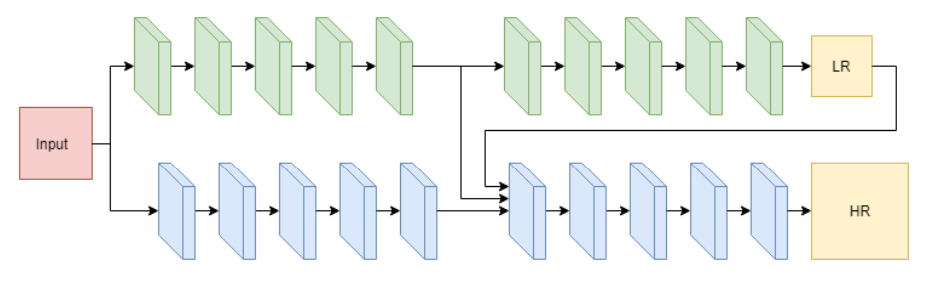$\hat{Z}_{i}=f_{l}\left(X_{i} ; \theta_{l}\right)$

$\hat{Y}_{i}=f_{h}\left(X_{i}, \hat{Z}_{i} ; \theta_{l}, \theta_{h}\right)$

$\mathcal{L}\left(\theta_{l}, \theta_{h}\right)=\frac{1}{n} \sum_{i=1}^{n}\left(\lambda_{l} L\left(\hat{Z}_{i}, Z_{i}\right)+\lambda_{h} L\left(\hat{Y}_{i}, Y_{i}\right)\right)$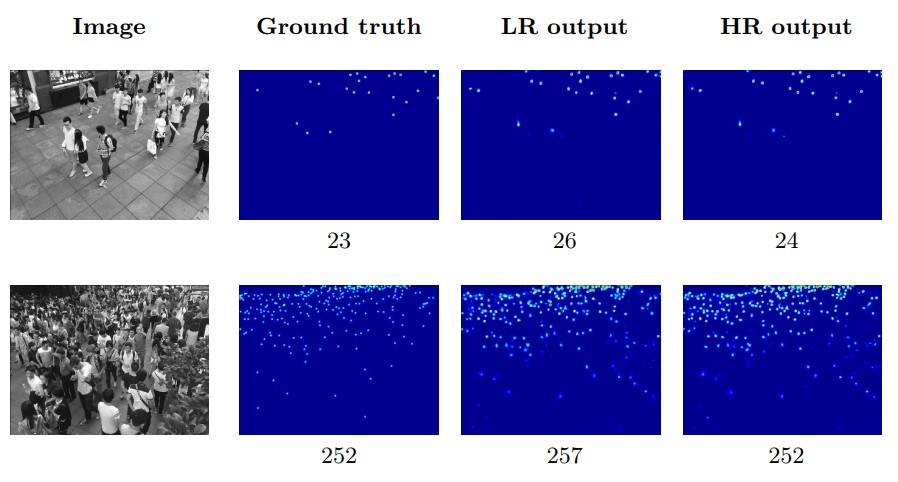### SANet(ECCV 2018)

Scale Aggregation Network for Accurate and Efficient Crowd Counting，该方法同样考虑要提取每个图像的多个尺度的人头信息，但并不采取类似MCNN的多阵列网络结构，其使用了类似于Inception架构的模块，在每个卷积层都同时使用不同大小的卷积核，最后通过反卷积得到最终的密度图。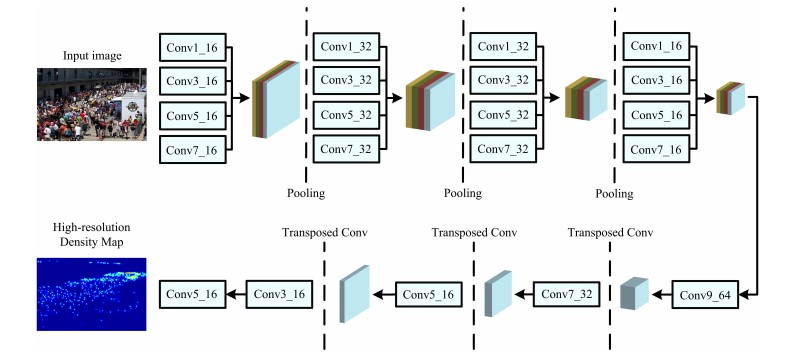### Summary

posted @ 2019-03-29 15:17  Brook_icv  阅读(9180)  评论(0编辑  收藏